# Cost curve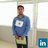"You Learn More From Failure Than From Success... Don’t Let It Stop You...!!! Failure Builds Character*... " em Chetu, Inc.
31 de Oct de 2015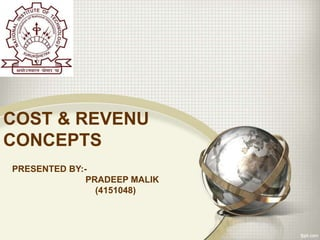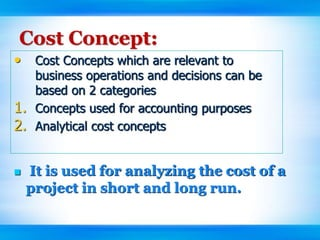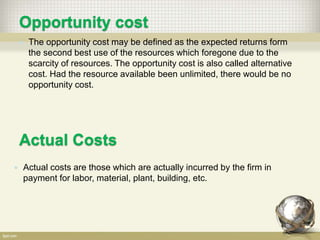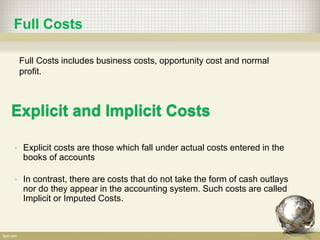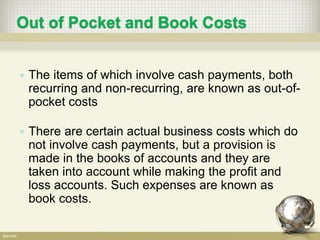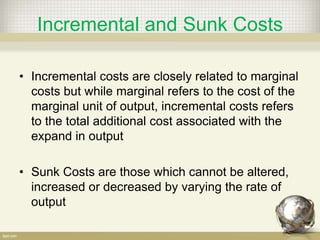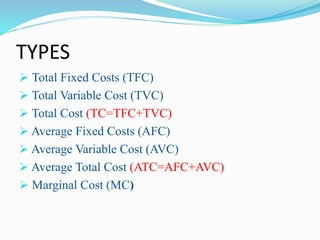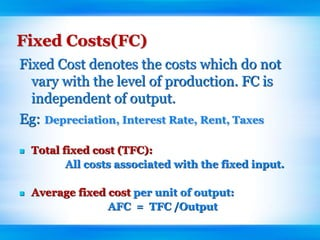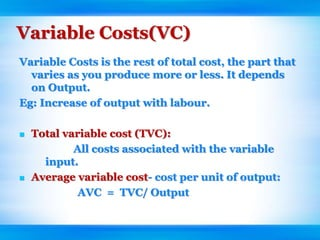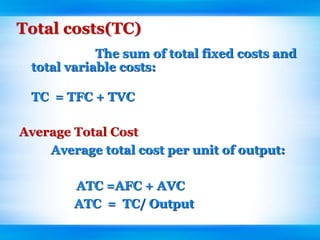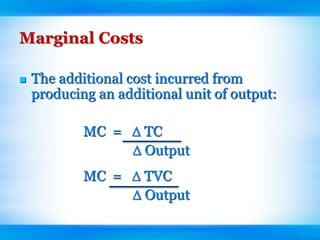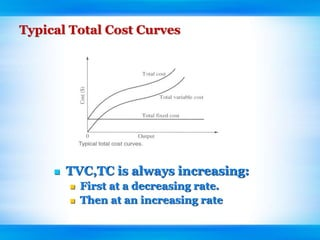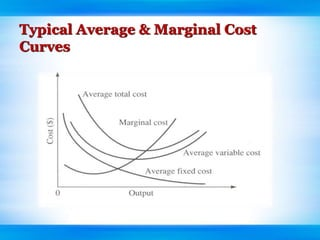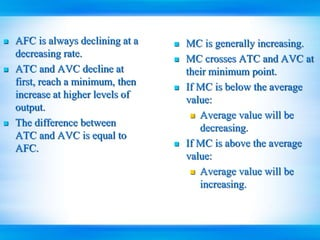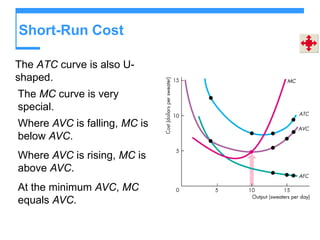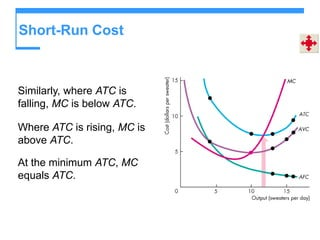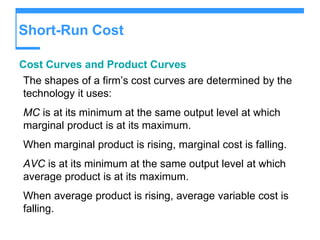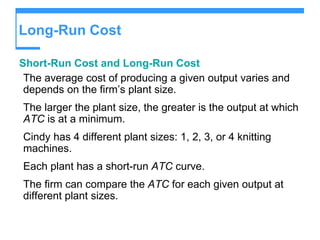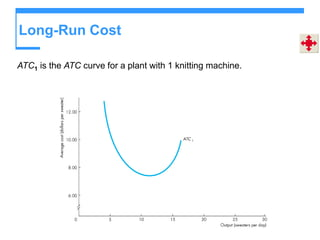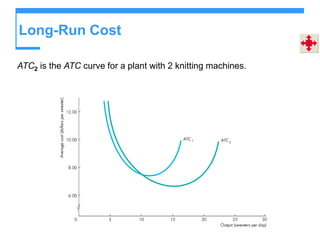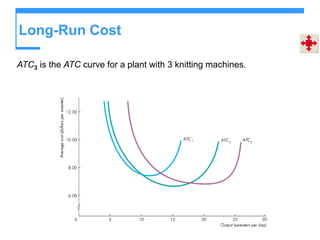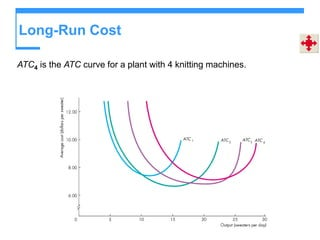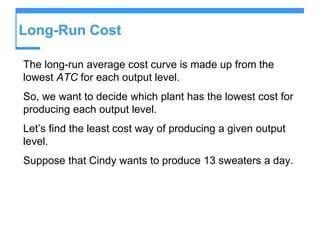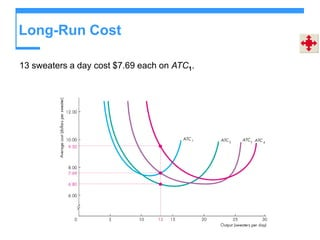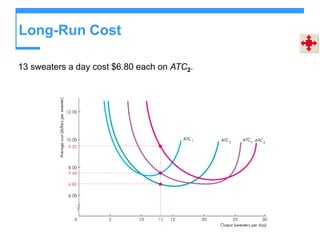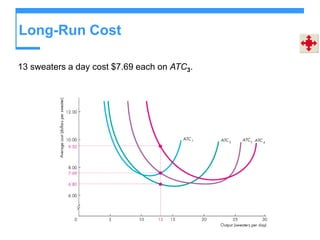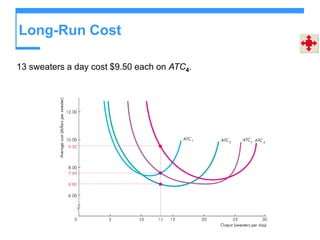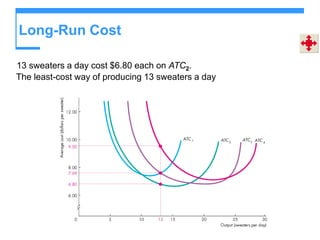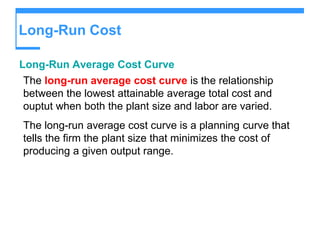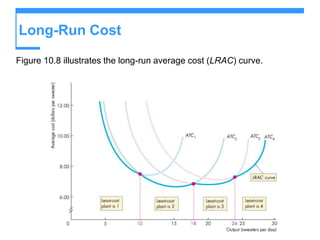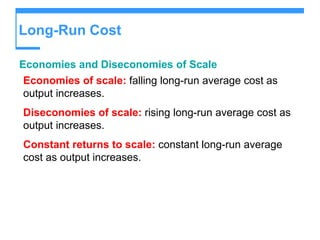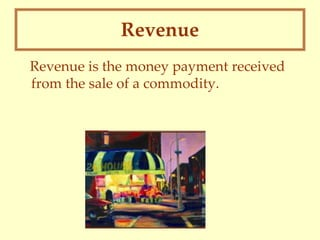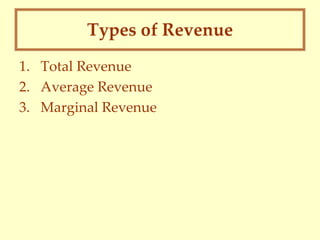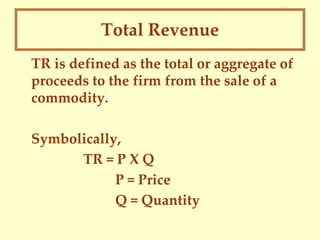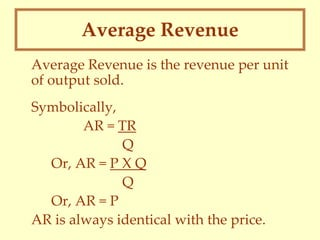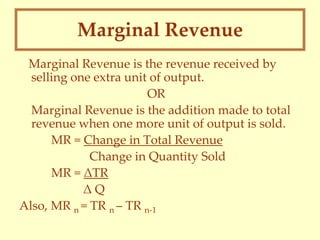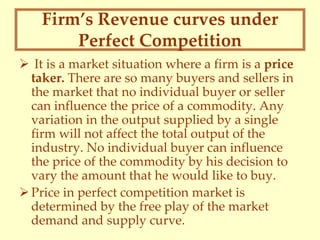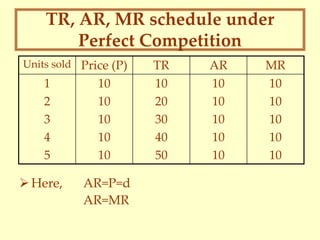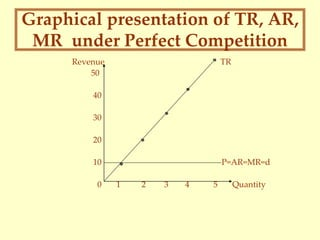1 de 40

### Cost curve

• 1. COST & REVENU CONCEPTS PRESENTED BY:- PRADEEP MALIK (4151048)
• 2. Cost Concept:  It is used for analyzing the cost of a project in short and long run. • Cost Concepts which are relevant to business operations and decisions can be based on 2 categories 1. Concepts used for accounting purposes 2. Analytical cost concepts
• 3. Opportunity cost  The opportunity cost may be defined as the expected returns form the second best use of the resources which foregone due to the scarcity of resources. The opportunity cost is also called alternative cost. Had the resource available been unlimited, there would be no opportunity cost. Actual Costs  Actual costs are those which are actually incurred by the firm in payment for labor, material, plant, building, etc.
• 4. Full Costs includes business costs, opportunity cost and normal profit. Full Costs Explicit and Implicit Costs  Explicit costs are those which fall under actual costs entered in the books of accounts  In contrast, there are costs that do not take the form of cash outlays nor do they appear in the accounting system. Such costs are called Implicit or Imputed Costs.
• 5. Out of Pocket and Book Costs  The items of which involve cash payments, both recurring and non-recurring, are known as out-of- pocket costs  There are certain actual business costs which do not involve cash payments, but a provision is made in the books of accounts and they are taken into account while making the profit and loss accounts. Such expenses are known as book costs.
• 6. Incremental and Sunk Costs • Incremental costs are closely related to marginal costs but while marginal refers to the cost of the marginal unit of output, incremental costs refers to the total additional cost associated with the expand in output • Sunk Costs are those which cannot be altered, increased or decreased by varying the rate of output
• 7.  Total Fixed Costs (TFC)  Total Variable Cost (TVC)  Total Cost (TC=TFC+TVC)  Average Fixed Costs (AFC)  Average Variable Cost (AVC)  Average Total Cost (ATC=AFC+AVC)  Marginal Cost (MC) TYPES
• 8. Fixed Costs(FC) Fixed Cost denotes the costs which do not vary with the level of production. FC is independent of output. Eg: Depreciation, Interest Rate, Rent, Taxes  Total fixed cost (TFC): All costs associated with the fixed input.  Average fixed cost per unit of output: AFC = TFC /Output
• 9. Variable Costs(VC) Variable Costs is the rest of total cost, the part that varies as you produce more or less. It depends on Output. Eg: Increase of output with labour.  Total variable cost (TVC): All costs associated with the variable input.  Average variable cost- cost per unit of output: AVC = TVC/ Output
• 10. Total costs(TC) The sum of total fixed costs and total variable costs: TC = TFC + TVC Average Total Cost Average total cost per unit of output: ATC =AFC + AVC ATC = TC/ Output
• 11. Marginal Costs  The additional cost incurred from producing an additional unit of output: MC =  TC  Output MC =  TVC  Output
• 12. Typical Total Cost Curves  TVC,TC is always increasing:  First at a decreasing rate.  Then at an increasing rate
• 13. Typical Average & Marginal Cost Curves
• 14.  AFC is always declining at a decreasing rate.  ATC and AVC decline at first, reach a minimum, then increase at higher levels of output.  The difference between ATC and AVC is equal to AFC.  MC is generally increasing.  MC crosses ATC and AVC at their minimum point.  If MC is below the average value:  Average value will be decreasing.  If MC is above the average value:  Average value will be increasing.
• 15. Short-Run Cost The ATC curve is also U- shaped. The MC curve is very special. Where AVC is falling, MC is below AVC. Where AVC is rising, MC is above AVC. At the minimum AVC, MC equals AVC.
• 16. Short-Run Cost Similarly, where ATC is falling, MC is below ATC. Where ATC is rising, MC is above ATC. At the minimum ATC, MC equals ATC.
• 17. Short-Run Cost Cost Curves and Product Curves The shapes of a firm’s cost curves are determined by the technology it uses: MC is at its minimum at the same output level at which marginal product is at its maximum. When marginal product is rising, marginal cost is falling. AVC is at its minimum at the same output level at which average product is at its maximum. When average product is rising, average variable cost is falling.
• 18. Long-Run Cost Short-Run Cost and Long-Run Cost The average cost of producing a given output varies and depends on the firm’s plant size. The larger the plant size, the greater is the output at which ATC is at a minimum. Cindy has 4 different plant sizes: 1, 2, 3, or 4 knitting machines. Each plant has a short-run ATC curve. The firm can compare the ATC for each given output at different plant sizes.
• 19. Long-Run Cost ATC1 is the ATC curve for a plant with 1 knitting machine.
• 20. Long-Run Cost ATC2 is the ATC curve for a plant with 2 knitting machines.
• 21. Long-Run Cost ATC3 is the ATC curve for a plant with 3 knitting machines.
• 22. Long-Run Cost ATC4 is the ATC curve for a plant with 4 knitting machines.
• 23. Long-Run Cost The long-run average cost curve is made up from the lowest ATC for each output level. So, we want to decide which plant has the lowest cost for producing each output level. Let’s find the least cost way of producing a given output level. Suppose that Cindy wants to produce 13 sweaters a day.
• 24. Long-Run Cost 13 sweaters a day cost \$7.69 each on ATC1.
• 25. Long-Run Cost 13 sweaters a day cost \$6.80 each on ATC2.
• 26. Long-Run Cost 13 sweaters a day cost \$7.69 each on ATC3.
• 27. Long-Run Cost 13 sweaters a day cost \$9.50 each on ATC4.
• 28. Long-Run Cost 13 sweaters a day cost \$6.80 each on ATC2. The least-cost way of producing 13 sweaters a day
• 29. Long-Run Cost Long-Run Average Cost Curve The long-run average cost curve is the relationship between the lowest attainable average total cost and ouptut when both the plant size and labor are varied. The long-run average cost curve is a planning curve that tells the firm the plant size that minimizes the cost of producing a given output range.
• 30. Long-Run Cost Figure 10.8 illustrates the long-run average cost (LRAC) curve.
• 31. Long-Run Cost Economies and Diseconomies of Scale Economies of scale: falling long-run average cost as output increases. Diseconomies of scale: rising long-run average cost as output increases. Constant returns to scale: constant long-run average cost as output increases.
• 32. Revenue Revenue is the money payment received from the sale of a commodity.
• 33. Types of Revenue 1. Total Revenue 2. Average Revenue 3. Marginal Revenue
• 34. Total Revenue TR is defined as the total or aggregate of proceeds to the firm from the sale of a commodity. Symbolically, TR = P X Q P = Price Q = Quantity
• 35. Average Revenue Average Revenue is the revenue per unit of output sold. Symbolically, AR = TR Q Or, AR = P X Q Q Or, AR = P AR is always identical with the price.
• 36. Marginal Revenue Marginal Revenue is the revenue received by selling one extra unit of output. OR Marginal Revenue is the addition made to total revenue when one more unit of output is sold. MR = Change in Total Revenue Change in Quantity Sold MR = ΔTR Δ Q Also, MR n = TR n – TR n-1
• 37. Firm’s Revenue curves under Perfect Competition  It is a market situation where a firm is a price taker. There are so many buyers and sellers in the market that no individual buyer or seller can influence the price of a commodity. Any variation in the output supplied by a single firm will not affect the total output of the industry. No individual buyer can influence the price of the commodity by his decision to vary the amount that he would like to buy. Price in perfect competition market is determined by the free play of the market demand and supply curve.
• 38. TR, AR, MR schedule under Perfect Competition Here, AR=P=d AR=MR Units sold Price (P) TR AR MR 1 2 3 4 5 10 10 10 10 10 10 20 30 40 50 10 10 10 10 10 10 10 10 10 10
• 39. Graphical presentation of TR, AR, MR under Perfect Competition Revenue TR 50 40 30 20 10 P=AR=MR=d 0 1 2 3 4 5 Quantity